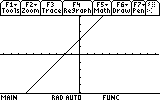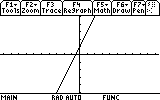# Plot a given equation on a graph

### How can a line or curve be represented algebraically?

A line can be expressed algebraically in several forms. The easiest to learn is called slope-intercept, and is written in the form of $$y=mx+b$$. The letters x and y represent the coordinates on the graph, m is the slope, and b is the y-intercept. The slope is the steepness of the line, and the y-intercept is where the line crosses the vertical axis. A slope of 5 is a very steep line, and a slope of 1/5 is almost flat. A y-intercept of 4 means that the line contains the point $$(0,4)$$, which is on the y-axis. Here is the graph of $$y=1x+2$$:As you can see, the line crosses the y-axis (vertical line) at the point $$(0,2)$$. You might also notice that for every point that the line moves right, it moves 1 point up, which is a slope of 1. The basic idea of a slope is that the amount you move up is on the top and the amount to move right is on the bottom. A slope of 1/2 means go up 1 and right 2, and a slope of 4 (or 4/1) means go up 4 for every 1 you go right. To understand slopes further, read our lesson on the slope of a line.

The easiest way to graph a line is to first plot the y-intercept. In the equation $$y=2x + 1$$, the y-intercept is 1, which means that the point $$(0,1)$$ is part of the line. Next, you should look at the slope. A slope of 3/1 means that you should go up 3 and right 1, giving you another point of $$(4,1)$$. Connect these two points and continue the line, and you're done!Another common way of writing lines involves using point-slope form. This way you are given one point and the slope to calculate the others. A line would be given like this in slope-intercept form: $$y - y_1 = m(x - x_1)$$

If you know the point $$(1,2)$$ is on the line and the slope is 1/2, you can easily write the line as $$y - 2 = \frac{1}{2}(x - 1)$$. To graph it, just start at the given point (1,2) and move up 1 and right 2. For more information on this subject you might want to continue browsing our site or perform a search on Google for graphing Lines. There are other lessons on graphing lines available on other websites